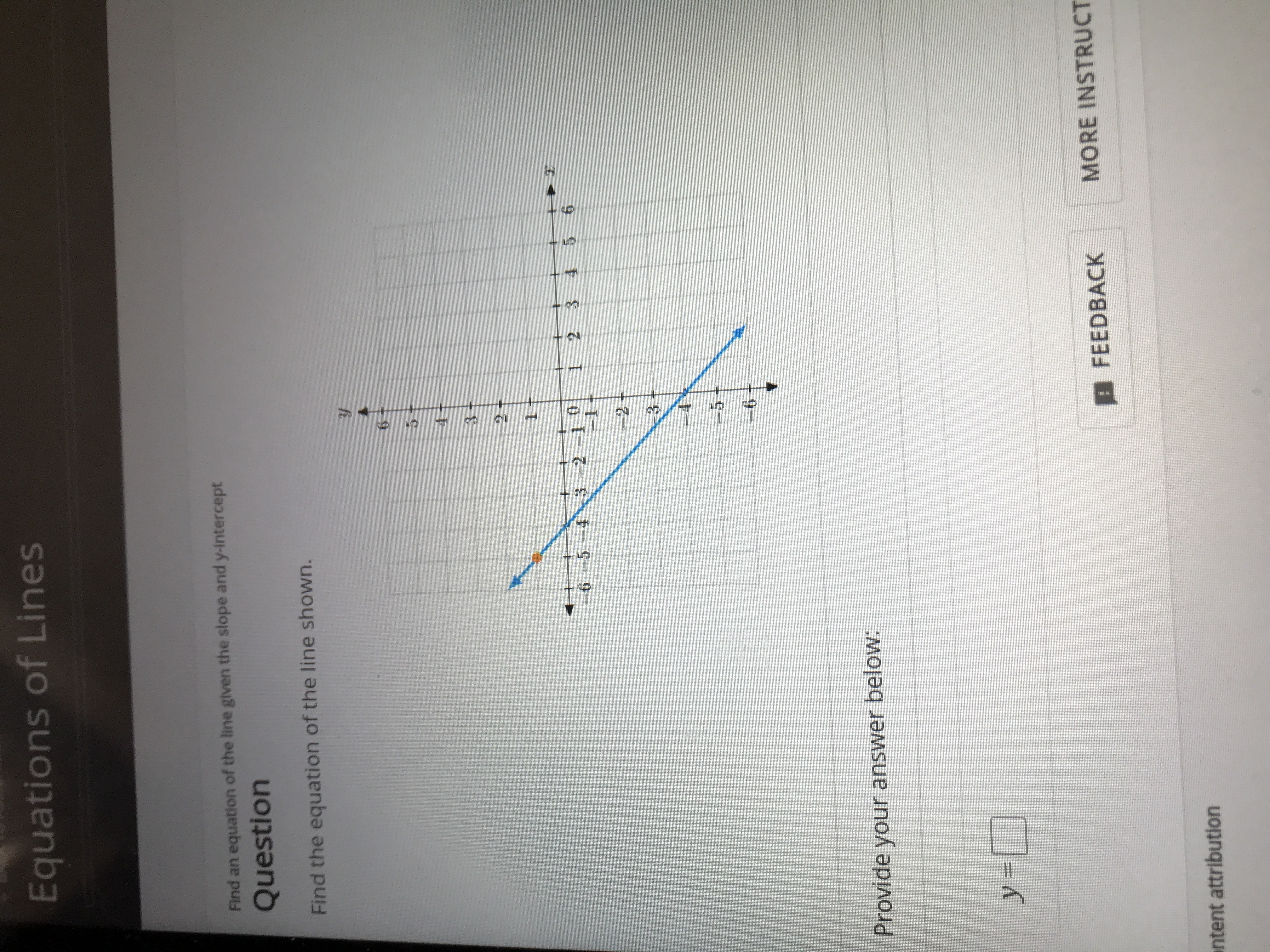# Equations of LinesFind an equation of the line given the slope and y-interceptQuestionFind the equation of the line shown.322 10652 336Provide your answer below:y=MORE INSTRUCTFEEDBACKntent attribution20

Question
66 viewshelp_outlineImage TranscriptioncloseEquations of Lines Find an equation of the line given the slope and y-intercept Question Find the equation of the line shown. 3 2 2 10 65 2 3 3 6 Provide your answer below: y= MORE INSTRUCT FEEDBACK ntent attribution 20 fullscreen
check_circle

Step 1

Let equation of the line be y=mx+c, where c is y-intercept...

### Want to see the full answer?

See Solution

#### Want to see this answer and more?

Solutions are written by subject experts who are available 24/7. Questions are typically answered within 1 hour.*

See Solution
*Response times may vary by subject and question.
Tagged in

### Algebra